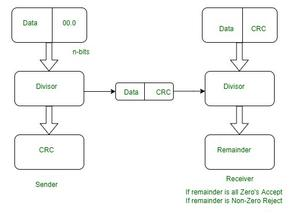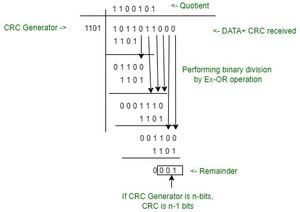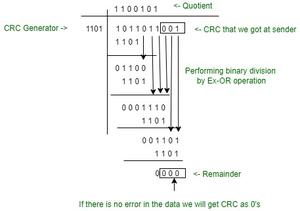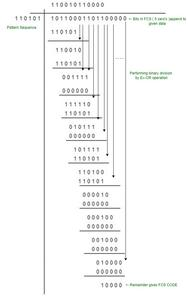Open in App
Not now

# What Is the Difference Between CRC and FCS?

• Last Updated : 25 Nov, 2021

Cyclic Redundancy Check(CRC): Cyclic redundancy check is generally called an error detection mechanism because the special numbers are appended with a block of data so that the changes that are being detected with respect to any changes introduced in the storage.
It is a more complex algorithm that is derived from simple CHECKSUM, MODULO ARITHMETIC, which is being treated out on each input word as it is a set of the coefficients for the polynomials.
CRC is commonly used in digital networks and storage devices to detect accidental changes to raw data. If CRC generator is n bits, CRC is (n-1) bits.

CRC working condition:

A CRC-enabled device calculates a short, fixed-length binary sequence, known as the check value or CRC, for each block of data to be sent or stored and appends it to the data, forming a code word. If the CRC values do not match, then the block contains a data error.CRC Working

Example:

Problem: Construct CRC message, let the divisor is 1101 and the data is 1011011.

Solution: As the length of the divisor is 4, we have to add 4-1=3 zeros to the end of the word.
Given data is 1011011 and CRC bits are 000 , i.e. 1011011000  {data + CRC received)

Sender side:Sender Side

So, the CRC, 001 is added to the message, 1011011.
The transmitted message is 1011011001The above example, explains that the sender sends the data to the receiver without errors, and the receiver receives the correct data.
Suppose if the receiver got a corrupted packet, the reminder will not get zero on the receiver side.

Frame Check Sequence: An FCS(Frame Check Sequence) is an error-detecting code that is being added to the frame in a protocol. Frames are used to send payload data from a source to a destination, that is this frame is being used to send the data to the application from the source to the destination.

• The FCS is the only one that provides error detection and recovery. It is performed on various separate means of the protocols.
• Generally, FCS is transmitted when the receiver is able to calculate the running sum of the entire frame together with most trailing FCS which are being expected to see the fixed result.
• The frame that ends with frame check sequence (FCS), helps in detecting in-transit corruption of data of 32-bit cyclic redundancy check.

Example:

Problem– Consider a message in bits of 1011 0001 1011 and a divisor of 110101 then find the Frame Check Sequence (FCS)?

Solution– If the divisor is n bits, we append n-1 bits at the end of the message. Here, the divisor is of 6 bits, so append 5 zeros at the end of the message and Perform XOR operation.
The given pattern sequence or divisor is 110101

Bits in FCS = number of bits pattern sequence-1
= 6-1
= 5 bits.

Consider the given data is 101100011011

Padding 5 zeros, because FCS bits are 5.

To find out the FCS code apply the cyclic redundancy check method by performing an XOR operation.

Note- In FCS When the MSB bit is zero we insert a zero bit sequence of the same length as the divisor and perform an XOR operation.FCS example

Therefore the frame check sequence is 1 0 0 0 0. The remainder gives the FCS code.

The transmitted sequence is message+ FCS Code
Therefore, the transmitted sequence is 10110001101110000

## Difference between CRC and FCS

My Personal Notes arrow_drop_up